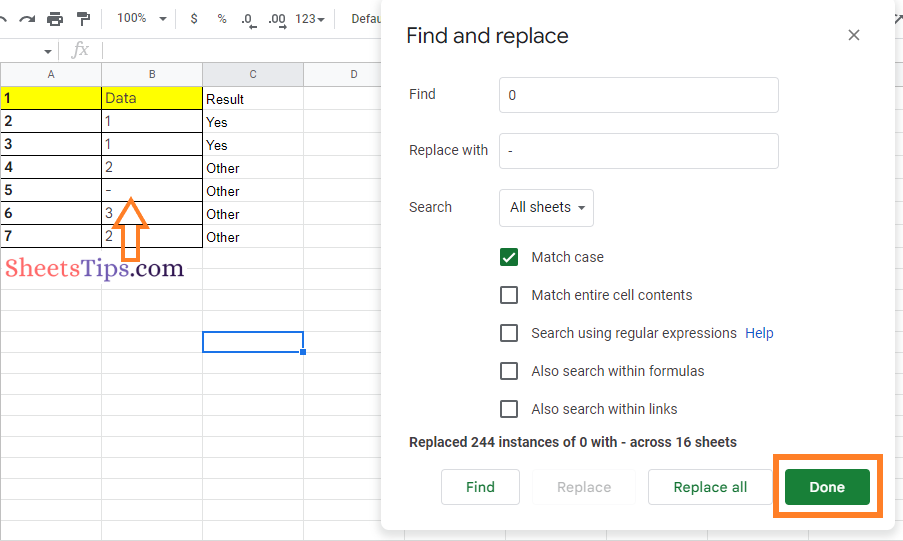# How To Show Zero as Dash in Google Sheets? – Replace Dash With Zero

Replace Dash with Zero in Google Sheets: When working with numerical data sets on the spreadsheet, it is quite often we face zero values in the spreadsheet. Most of us, however, would like to distinguish zero values from other values by using symbols such as dash (-), nil, and many others. Differentiating Zero from other values will assist spreadsheet users in gaining more insight into the data. Separating the zero values from others is extremely important, And to get this done, Google Sheets allows its users to change the Zero to a Dash using various methods.

In this article, we will explain the various methods with the help of which we can replace 0 as a dash in the spreadsheet using Google Sheets Tips. Scroll down to find out more.

## How Do You Put a Dash Instead of 0 Using Custom Format Number?

As discussed above, there are several methods with the help of which we can simply replace the Zero as a Dash in Google Sheets. One of the finest methods which help to replace the zero with a dash is by using the Custom number format option. The detailed steps for changing Zero to Dash in the spreadsheet using Custom number formats are highlighted below:

• 2nd Step: Now on the homepage, click on the “Format” tab and choose “Number” from the drop-down menu.
• 3rd Step: From the Number sub-drop down menu, choose the “Custom number format” option.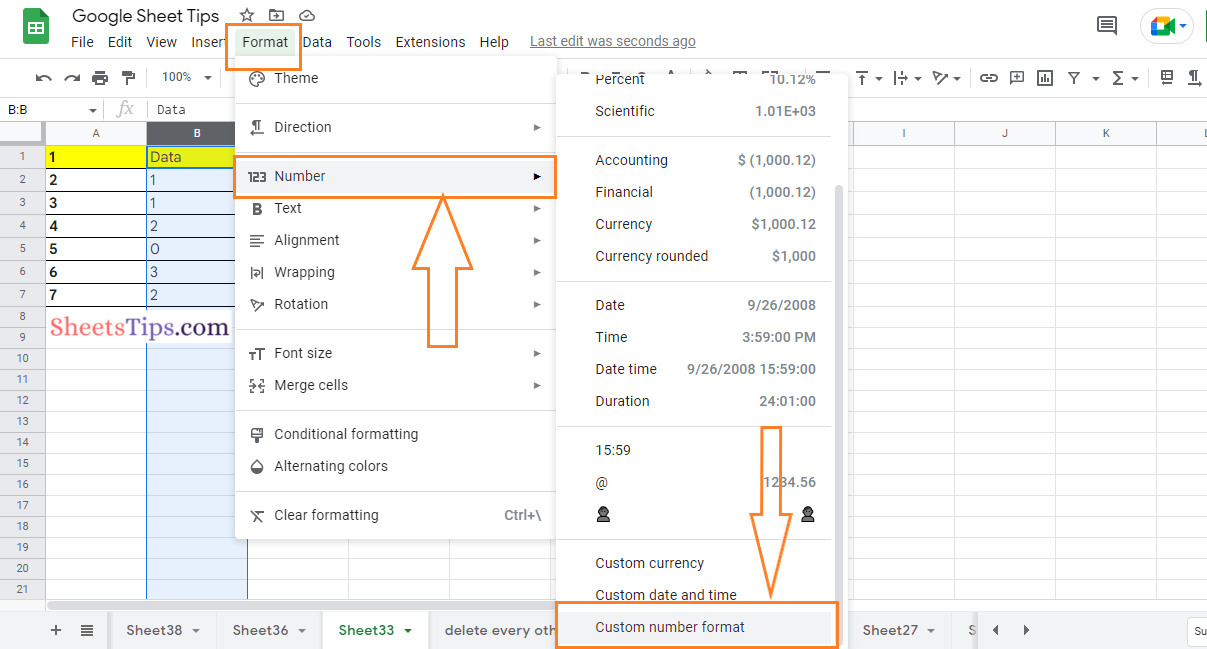• 4th Step: In the “Custom number format” box, enter the following code “#,##0; [red]#,##0;-” and you will see the image as shown below.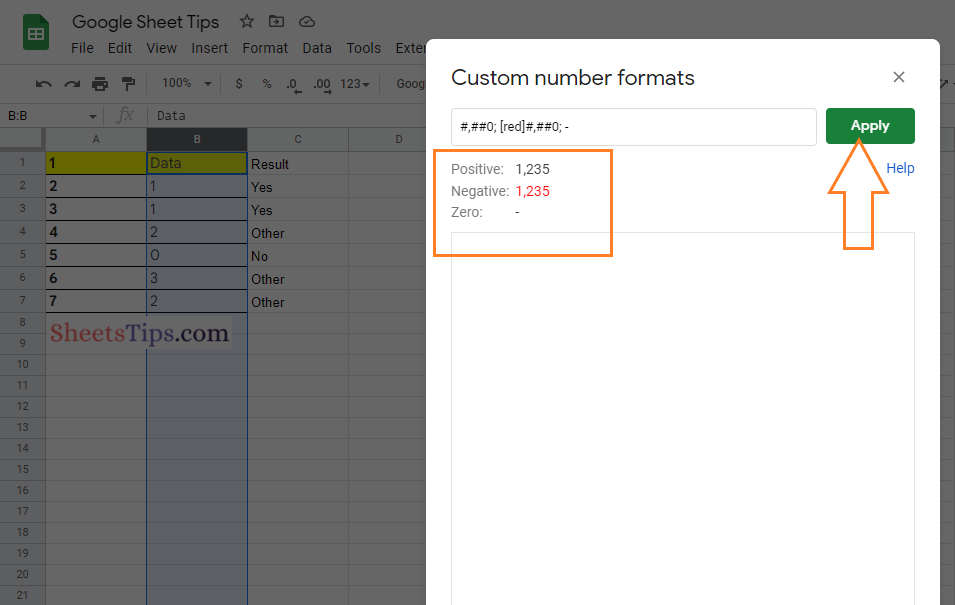• 5th Step: Press the “Apply” button and you will see the “Zero” being replaced by a dash as shown in the image below.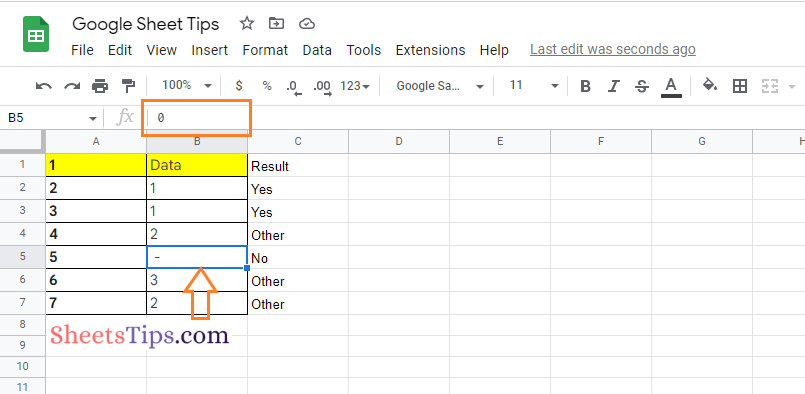### Using the TEXT Function to Show Zero as a Dash in Google Sheets

Google Sheets also allows its users to show the Zero as a Dash using the TEXT function. The detailed steps on how to get this done are outlined below.

• 2nd Step: Now on the homepage, move to the cell where you want to convert the zeros into dashes.
• 3rd Step: Enter the formula “=TEXT(B5,” #, ##0; #, ##0;-“). Here, B5 is the cell range. Based on your cell range, you can modify the formula.
• 4th Step: Press the “Return” key and you will find the results as shown in the image given below.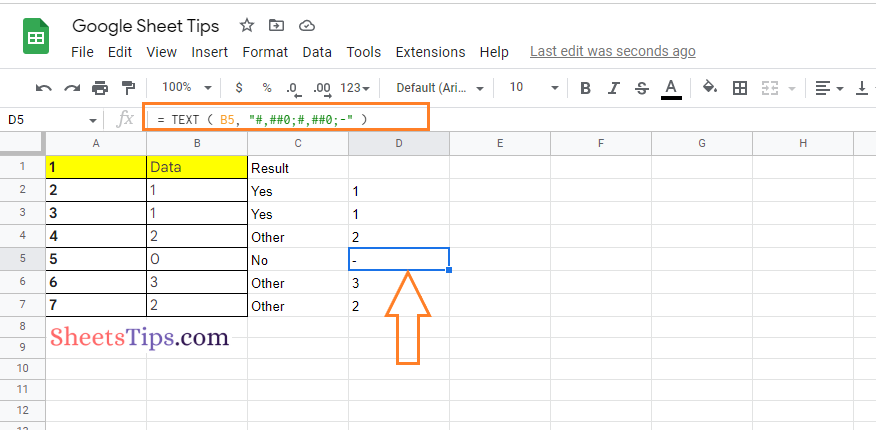### Using the QUERY Function to Replace Zero with a Dash

The steps to replace Zero with Dash using the QUERY function in the Google Sheets are given below:

• 2nd Step: Now on the homepage, move to the desired cell to apply the formula.
• 3rd Step: Type the formula “=QUERY(B2:B7,”format B'”; #, ##0; [red] #, ##0; ‘,”0)“. Here, B is the cell range. You can alter the cell range according to your preference.
• 4th Step: Press the “Return” key and you will find the results as shown in the image below.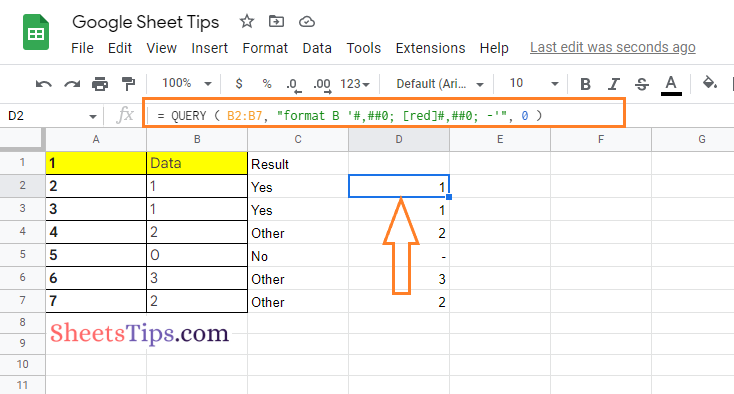### Using the IF Function to Display a Dash Instead of Zero

The IF function in Google Sheets allows users to display the dash instead of zero. The steps to use the IF function in the spreadsheet are given below:

• 1st Step: Open the Google Sheets to replace the dash with Zero.
• 2nd Step: Now on the homepage, move to the cell where you want to show the results.
• 3rd Step: Here enter the formula “= If (B2-C2 = 0,”-,” B2-C2″)“.
• 4th Step: Press the “Enter” key and you will find the results as shown in the image given below.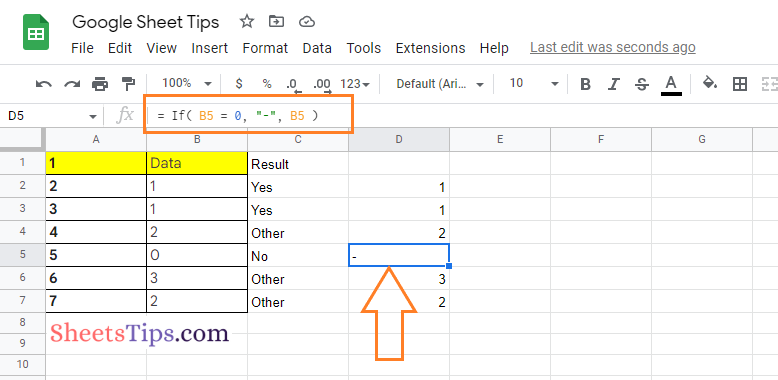### Using the Find and Replace Option to Show Dash Instead of Zero

Another easy method to replace a dash with a zero is to use the Find and Replace method. The detailed steps on how to use the Find and Replace method to replace a dash with zero are given below: